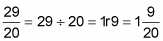##### Basic Math & Pre-Algebra All-in-One For Dummies (+ Chapter Quizzes Online)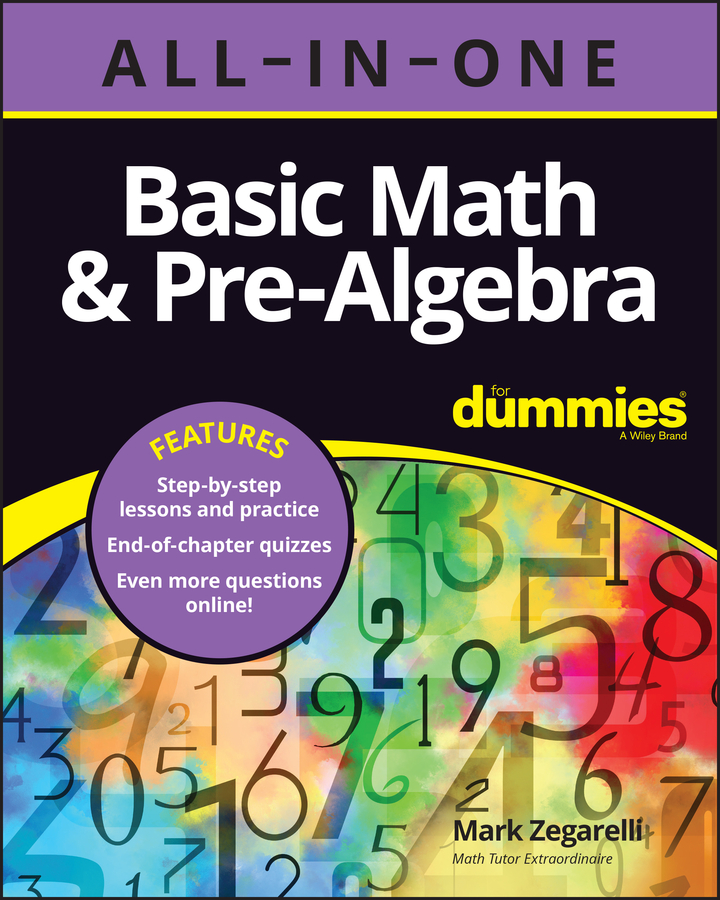When the fractions that you want to add have different denominators, there are a few different ways you can do it.

## Add fractions the easy way

At some point in your life, some teacher somewhere told you these golden words of wisdom: “You can’t add two fractions with different denominators.” Your teacher was wrong! You can use the easy way when the numerators and denominators are small (say, 15 or under).

Here’s the way to do it:

1. Cross-multiply the two fractions and add the results together to get the numerator of the answer.

Suppose you want to add the fractions 1/3 and 2/5. To get the numerator of the answer, cross-multiply. In other words, multiply the numerator of each fraction by the denominator of the other: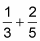1*5 = 5

2*3 = 6

Add the results to get the numerator of the answer:

5 + 6 = 11

2. Multiply the two denominators together to get the denominator of the answer.

To get the denominator, just multiply the denominators of the two fractions:

3*5 = 15

The denominator of the answer is 15.

3. Write your answer as a fraction.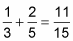When you add fractions, you sometimes need to reduce the answer that you get. Here’s an example: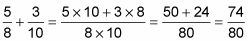Because the numerator and the denominator are both even numbers, you know that the fraction can be reduced. So try dividing both numbers by 2: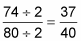This fraction can’t be reduced further, so 37/40 is the final answer.

In some cases, you may have to add more than one fraction. The method is similar, with one small tweak.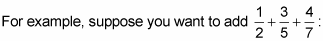1. Start out by multiplying the numerator of the first fraction by the denominators of all the other fractions.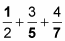(1*5*7) = 35

2. Do the same with the second fraction and add this value to the first.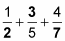35 + (3*2*7) = 35 + 42

3. Do the same with the remaining fraction(s).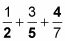35 + 42 + (4*2*5) = 35 + 42 + 40 = 117

When you’re done, you have the numerator of the answer.

4. To get the denominator, just multiply all the denominators together: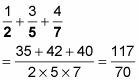You may need to reduce or change an improper fraction to a mixed number. In this example, you just need to change to a mixed number: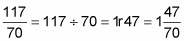## Add fractions with the quick trick method

You can’t always use this method, but you can use it when one denominator is a multiple of the other. Look at the following problem: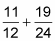First, solve it the easy way: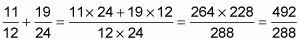Those are some big numbers, and you’re still not done because the numerator is larger than the denominator. The answer is an improper fraction. Worse yet, the numerator and denominator are both even numbers, so the answer still needs to be reduced.

With certain fraction addition problems, there is a smarter way to work. The trick is to turn a problem with different denominators into a much easier problem with the same denominator.

Before you add two fractions with different denominators, check the denominators to see whether one is a multiple of the other. If it is, you can use the quick trick:

1. Increase the terms of the fraction with the smaller denominator so that it has the larger denominator.

Look at the earlier problem in this new way:As you can see, 12 divides into 24 without a remainder. In this case, you want to raise the terms of 11/12 so that the denominator is 24: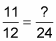To fill in the question mark, the trick is to divide 24 by 12 to find out how the denominators are related; then multiply the result by 11:

? = (24 ÷ 12) 11 = 22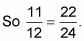2. Rewrite the problem, substituting this increased version of the fraction, and add.

Now you can rewrite the problem this way: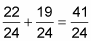As you can see, the numbers in this case are much smaller and easier to work with. The answer here is an improper fraction; changing it into a mixed number is easy: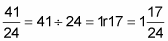Use the traditional way only when you can’t use either of the other methods (or when you know the least common multiple [LCM] just by looking at the denominators).

Here’s the traditional way to add fractions with two different denominators:

1. Find the LCM of the two denominators.

Suppose you want to add the fractions 3/4 + 7/10. First find the LCM of the two denominators, 4 and 10. Here’s how to find the LCM using the multiplication table method:

• Multiples of 10: 10, 20, 30, 40

• Multiples of 4: 4, 8, 12, 16, 20

So the LCM of 4 and 10 is 20.

2. Increase the terms of each fraction so that the denominator of each equals the LCM.

Increase each fraction to higher terms so that the denominator of each is 20.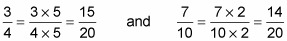3. Substitute these two new fractions for the original ones and add.

At this point, you have two fractions that have the same denominator: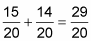When the answer is an improper fraction, you still need to change it to a mixed number: